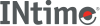﻿ psd (SDM)INtime SDK Help
psd (SDM)

Displays and lets you modify a field from a page directory entry. Use the pdd command to display one or more complete entries.

```psd (index).component
```

#### Parameters

`(index)`
The directory entry to modify. The first entry is index 0.
`.component`
The field in the entry to modify, from the following list:
 `ADDR` Base address of the second-level page table. `AV` Available bits for use by the OS. `S` (Pentium-specific) Size bit: 0 means 4 KB page. `A` Accessed bit: 1 means read or write to the page table. `PCD` Page-level Cache Disable bit: 1 means caching is disabled. `PWT` Page-level WriteThrough bit: 1 is writethrough, 0 is writeback. `U` User/Supervisor bit: 1 is application code and data, 0 is OS memory. `W` Read/Write bit: 1 is read/write, 0 is read-only. `P` Present bit: 1 means the page is present in memory.

#### Examples

Use the following commands to display the sixth entry (index 5) of the page directory and change the accessed bit of that entry.

```..pdd(5t)
PDIR(5T) ADDR=006c1000 AV=0 S=0 A=1 PCD=0 PWT=0 U=1 W=1 P=1
..psd(5t).a
1 - 0
..pdd(5t)
PDIR(5T) ADDR=006c1000 AV=0 S=0 A=0 PCD=0 PWT=0 U=1 W=1 P=1
```